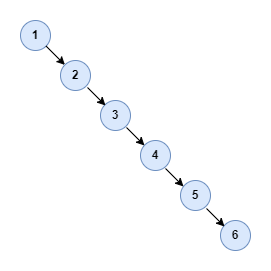# Understand Red Black Tree: part one - background

### Introduction

In this series of articles, I want to examine an important data structure: the red-black tree. The red-black tree is an advanced data structure that is difficult to fully understand. Maybe you have some wonders and confusion about it as follows:

• What is the meaning of red and black here?
• The red-black tree is known as a self-balancing binary search tree. But what’s the difference between it and others?
• Where is it used or applied?

• Why do we need the red-black tree and what is its advantage?
• How does a red-black tree work?
• How to write a red-black tree from scratch?

### Background of Red-Black Tree

In this section, let’s review the history of the red-black tree. During this process, I will show you why it was invented and what kind of advantages it can provide compared with other tree data structures.

First thing first, let’s define the red-black tree as follows:

• Red-Black Tree is a self-balancing binary search tree.

Let’s analyze this definition step by step:

• Tree: A tree is a nonlinear data structure, compared to arrays, linked lists, stacks and queues which are linear data structures. A tree is a data structure consisting of one node called the root and zero or one or more subtrees. One disadvantage of linear data structures is the time required to search a linear list is proportional to the size of the data set, which means that the time complexity is O(n). That’s why more efficient data structures like trees are invented to store and search data.

• General Tree: A general tree is a tree where each node may have zero or more children.

• Binary Tree: A binary tree is a specialized case of a general tree, where each node can have no more than two children.

• Binary Search Tree: A binary tree satisfying the binary search property is called a binary search tree(BST). To build a BST, the node with a key greater than any particular node is stored on the right sub-trees and the one equal to or less than is stored on the left sub-tree.

The average search time complexity of BST is O(logN), but in the worst case, it will be degraded to O(N). It happens when we insert the nodes one by one in order. For example, if we insert the elements in array [1, 2, 3, 4, 5, 6] into RST in order, what we get is as follows:This kind of unbalanced BST is degraded to a single linked list. So the BST needs to keep balanced during the insert and delete of the node. That’s where the self-balancing binary search tree comes from.

• Self-Balancing Binary Search Tree: it’s a binary search tree that automatically keeps its height small in the face of arbitrary item insertions and deletions. And the red-black tree is just one type of it.

### Summary

As the first post of this series, I examined the background of the red-black tree bit by bit. I hope you can understand what’s self-balancing binary search tree and why we need it. In the next post, I will start to examine the behavior and property of the red-black tree.